Ex 5.4

Chapter 5 Class 8 Squares and Square Roots
Serial order wise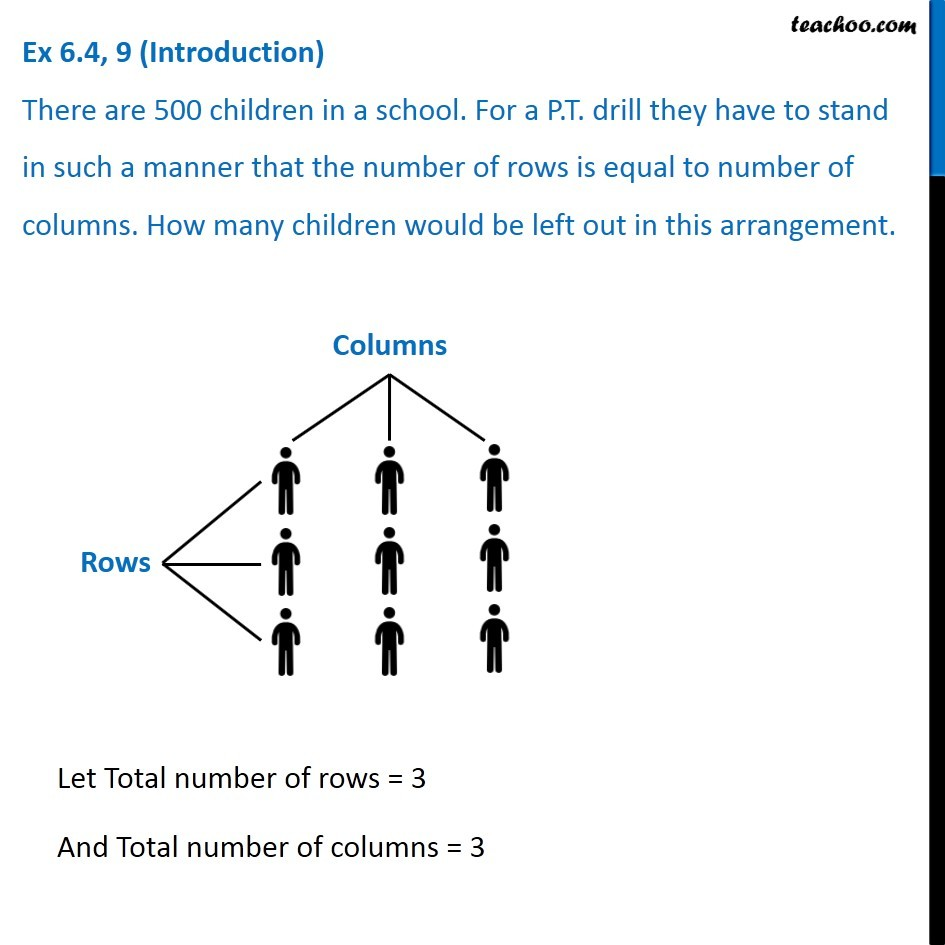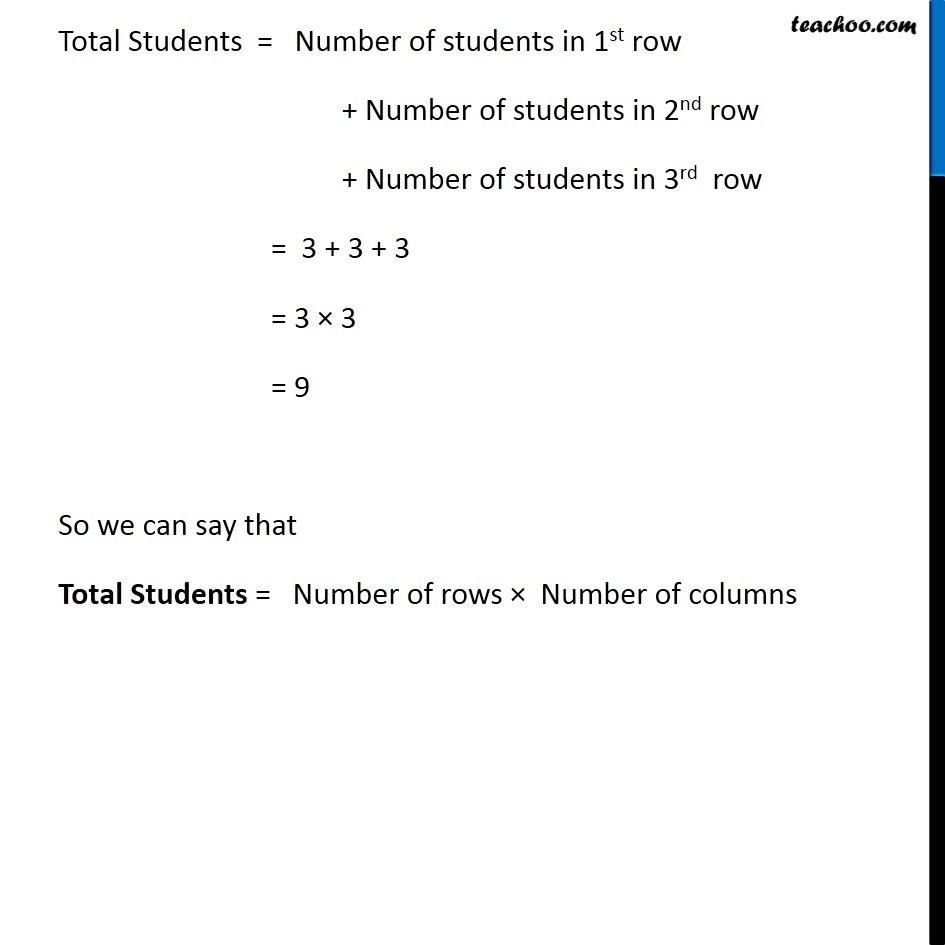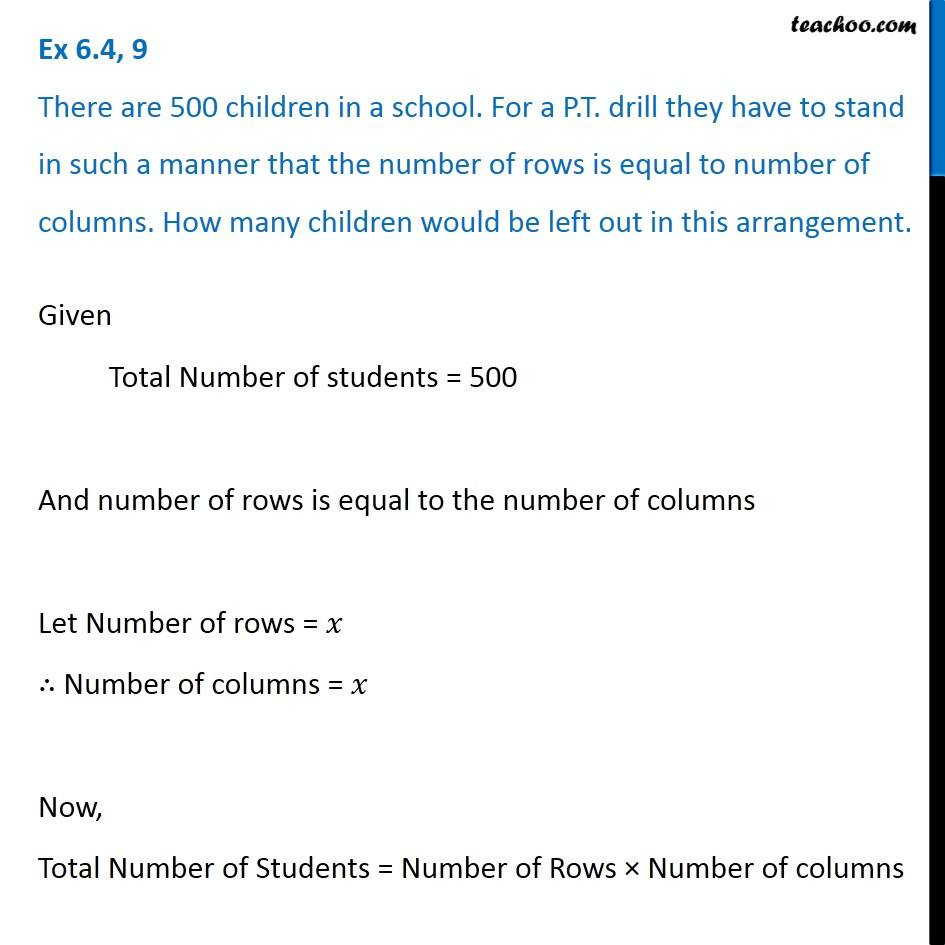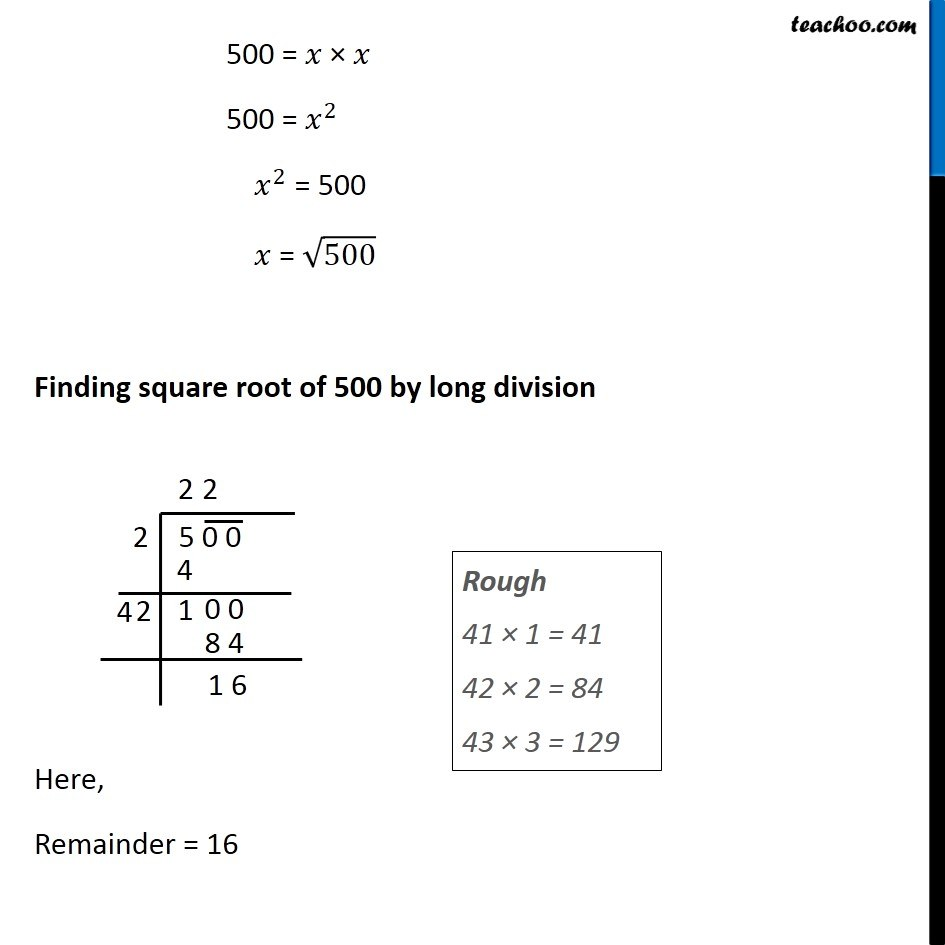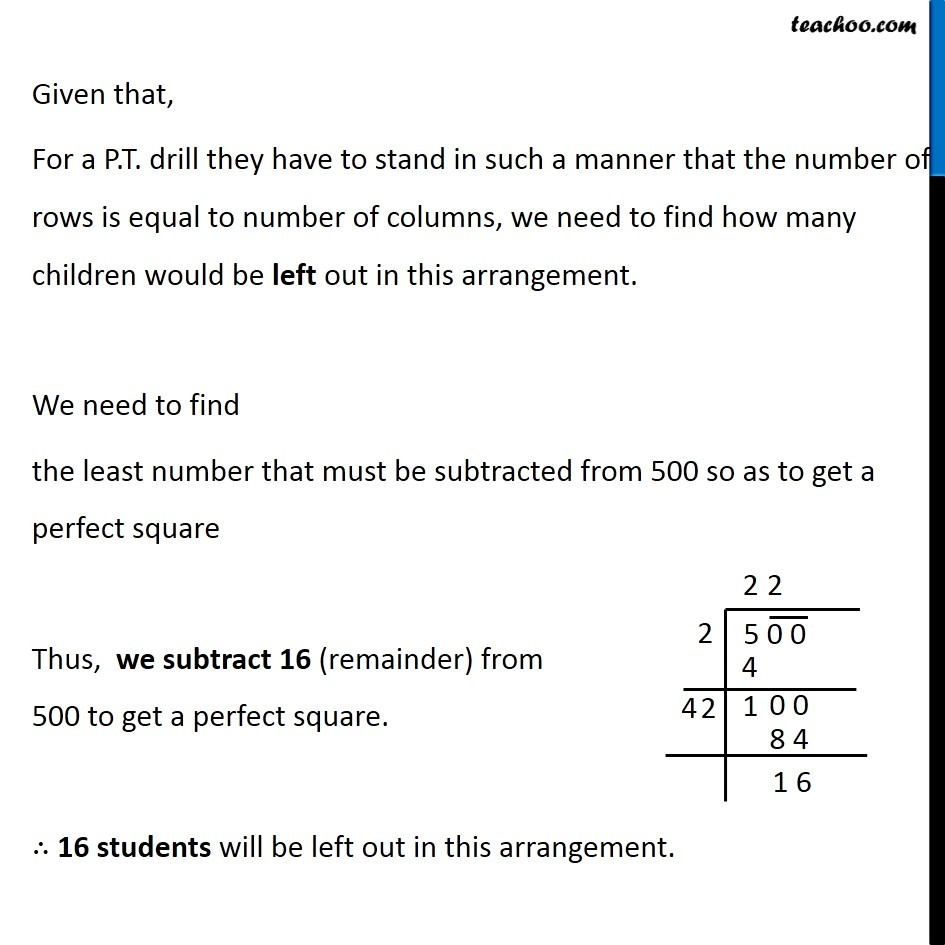Learn in your speed, with individual attention - Teachoo Maths 1-on-1 Class

### Transcript

Ex 5.4, 9 (Introduction) There are 500 children in a school. For a P.T. drill they have to stand in such a manner that the number of rows is equal to number of columns. How many children would be left out in this arrangement. Let Total number of rows = 3 And Total number of columns = 3 Total Students = Number of students in 1st row + Number of students in 2nd row + Number of students in 3rd row = 3 + 3 + 3 = 3 × 3 = 9 So we can say that Total Students = Number of rows × Number of columns Ex 5.4, 9 There are 500 children in a school. For a P.T. drill they have to stand in such a manner that the number of rows is equal to number of columns. How many children would be left out in this arrangement.Given Total Number of students = 500 And number of rows is equal to the number of columns Let Number of rows = 𝑥 ∴ Number of columns = 𝑥 Now, Total Number of Students = Number of Rows × Number of columns 500 = 𝑥 × 𝑥 500 = 𝑥^2 𝑥^2 = 500 𝑥 = √500 Finding square root of 500 by long division Here, Remainder = 16 Rough 41 × 1 = 41 42 × 2 = 84 43 × 3 = 129 Given that, For a P.T. drill they have to stand in such a manner that the number of rows is equal to number of columns, we need to find how many children would be left out in this arrangement. We need to find the least number that must be subtracted from 500 so as to get a perfect square Thus, we subtract 16 (remainder) from 500 to get a perfect square. ∴ 16 students will be left out in this arrangement.